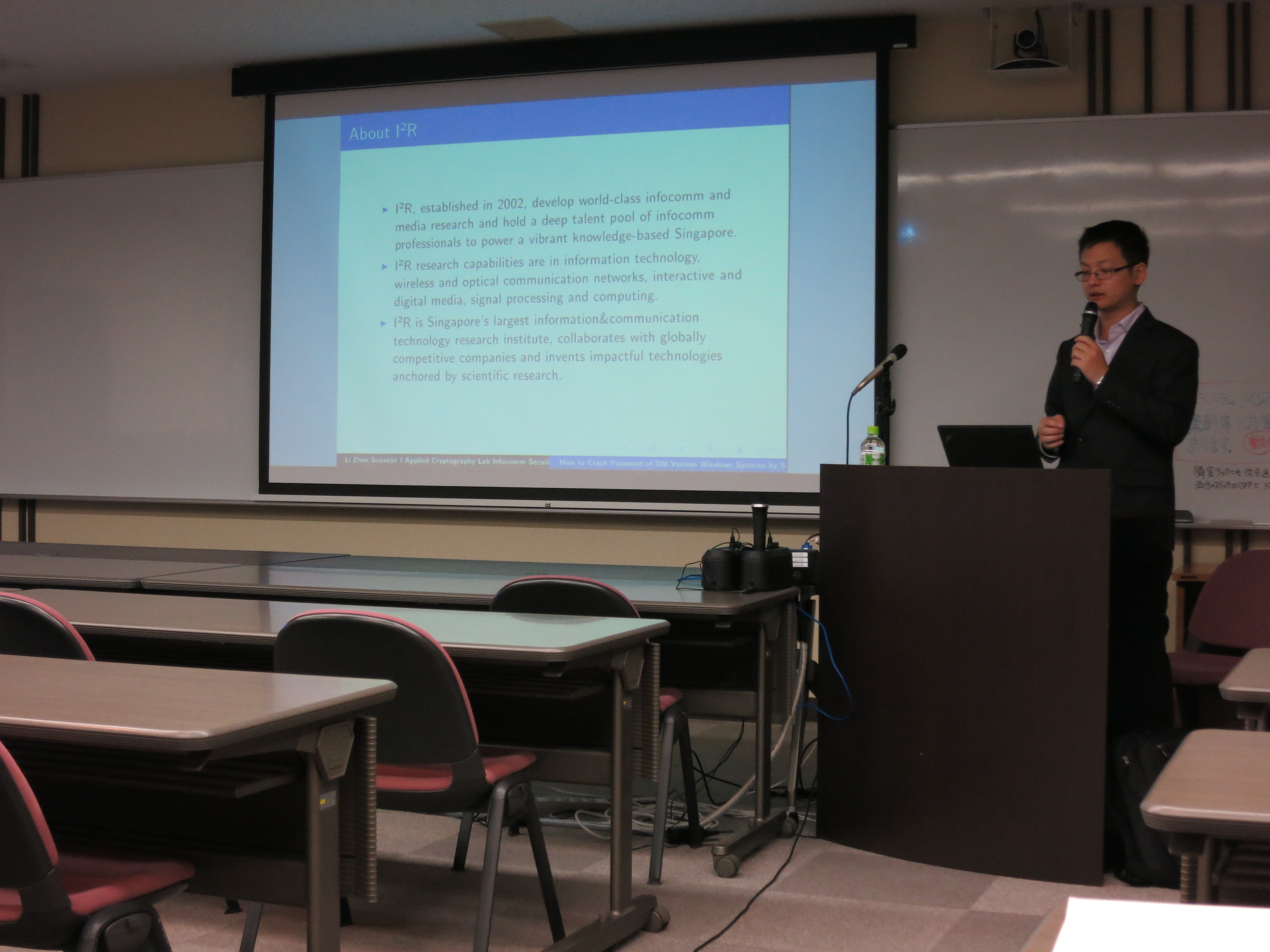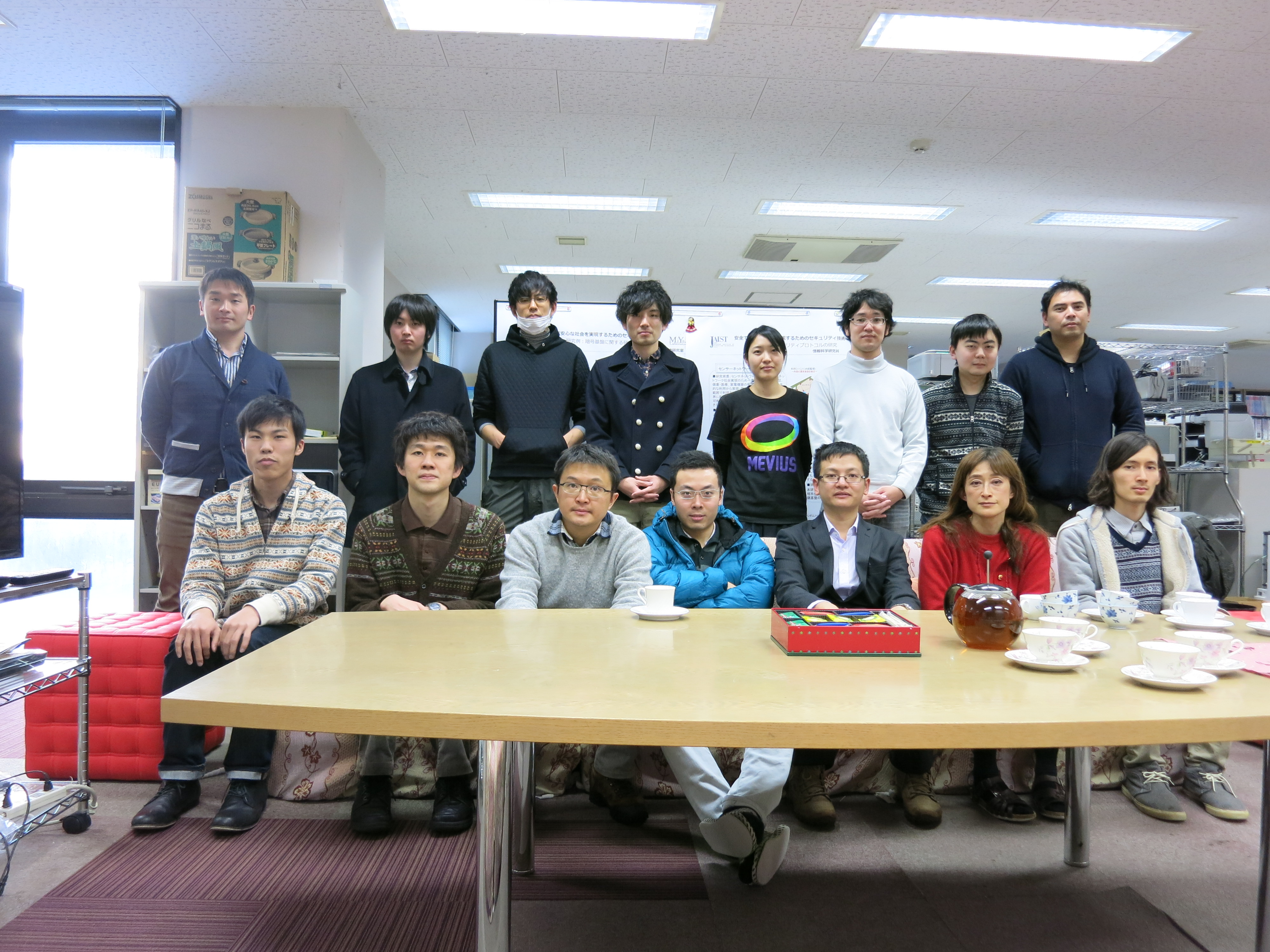日時：　平成26年12月23日 14時00分-16時00分場所：　情報科学研究科 5階 コラボレーションルーム7講演者氏名： Li Zhen
Institute for Infocomm Research, Singapore講演題目： How to Crack Password of Old Version Windows Systems by Solving Equations

In cryptanalysis and computer security, password cracking is the process of recovering passwords from data that have been stored in or transmitted by a computer system. The purpose of password cracking might be to gain unauthorized access to a system or to help a user recover a forgotten password.
Windows-based computers utilize several protocols to authenticate, including LAN Manager (LM), NT LAN Manager protocol (NTLM). A hash is the result of a cryptographic function that takes an arbitrarily sized string of data, performs a mathematical encryption function on it, and returns a fixed-size string. The LAN Manager hash is a password hashing algorithm which is used by earlier versions of Windows operating systems, while NTLM is used in newer versions such as Windows 2000, XP, Vista, and 7.
In order to crack Windows passwords we must first obtain the hashes stored within the operating system. The resulting hash is very different even if a slight change is made to the password. These hashes are stored in the Windows SAM file. This file is located on your system both at C:\Windows\System32\config, and also in the registry HKEY LOCAL MACHINEnSAM, but they are not accessible while the operating system has been booted. There are a few different options available to access the hash value in the computer, e.g. physical access, console access through remote desktop or VNC, network access, etc. A simple method is to reboot the computer into Linux from a flash drive, and then run a program that extracts passwords from the hashes.
After the access to the Windows password hash value, we can use several methods to reverse the hash value to the password of the Windows user. Although Windows password is based on DES and MD4 which are well-studied block cipher based hash functions, they still have several weaknesses in their designs. The older LM hash is more obvious in its weakness, where passwords longer than 7 characters are divided into two pieces and each piece is hashed separately. This weakness allows each half of the password to be attacked separately at exponentially lower cost than the whole, as only $$69^7 \approx 24^3$$ different 7-character password pieces are possible with the same character set. In addition, the LM hash does not use cryptographic salt, a standard technique to prevent pre-computed dictionary attacks. The newer NTLM v1 still uses DES as the hash function, while NTLM v2 uses MD4 to enhance the security, but again the state-of-the-art hash functions such as SHA-256 are not used.
Most methods of password cracking require the computer to produce many candidate passwords, each of which is checked. One example is brute-force cracking, in which a computer tries every possible key or password until it succeeds. In case of LM hash crack, there are only $$2 \times 69^7 \approx 2^{44}$$ combinations, but a single brute force search will take several hours based on computational efficient hardware such as GPGPU and FPGA. More common methods of password cracking, such as dictionary attacks, attempt to reduce the number of trials required and will usually be more efficient than brute force. Pre-computation of many candidates can be stored using a large memory, and the crack is performed by table lookup. In 1980, Hellman introduced a time-memory tradeoff, known as Hellman table cryptanalysis. In 2003, Ophcrack, an implementation of time-memory tradeoff (TMTO) technique was developed. It specifically targets the weaknesses of LM encryption, and includes precomputed data sufficient to crack an alphanumeric LM hash in a few seconds if it is found in the precomputed table. However, TMTO usually takes a lot of pre-computation time and typically needs several terabytes to store. In addition, dictionary attacks are achieved by time-memory tradeoff which will fail in crack occasionally.
In this work, an efficient equation solving method is presented for hash value cracking, which is an improved version of the basic characteristic set algorithm in a finite field. We analyzed and implemented the polynomial equation systems of binary case, called monic triangular set algorithm. Basic terminologies and operations of binary polynomial equation systems are introduced, and then the detailed algorithm is elaborated. Examples are illustrated step by step to make the students easy to follow. An improved zero decomposition algorithm is presented which can be used to reduce the zero set of an equation system to the union of zero sets of monic triangular sets. Specifically, the basic zero decomposition algorithm only checks for the existence of 1 in the polynomial set for termination, while the improved algorithm additionally checks whether 1 is the sum of any polynomials to invoke the termination earlier. In addition, linearization is applied to reduce the degree of the polynomials to improve efficiency.
To solve general systems of polynomial equations in $$n$$ variables, $$x_1, x_2,\ldots, x_n$$ are represented over binary field $$\mathbb F_2$$ in the form: \begin{align*} {\mathbb P}=\left\{% \begin{array}{lc@{\kern2pt}c@{\kern2pt}r} p_1(x_1,\ldots,x_n)&=&0,\\ p_2(x_1,\ldots,x_n)&=&0, \\ &\ldots& \\ p_k(x_1,\ldots,x_n)&=&0 \end{array}\right. \end{align*} which is $k$ equations of polynomials, and $x_1,\ldots,x_n$ are the binary variables of the Windows password. Our goal is to apply some algorithms on $\mathbb P$ to obtain triangular sets $\mathbb T_1, \mathbb T_i,\ldots, \mathbb T_m$, such that ${\rm Zero}_q(\mathbb P) = \bigcup^m_{i=1}{\rm Zero}_q(\mathbb T_i)$ with ${\rm Zero}_q(\mathbb T_i)$ being disjoint. A triangular set of polynomials is a set of polynomials in which the classes of all the polynomials are different: \begin{align*} {\mathbb T}=\left\{% \begin{array}{lc@{\kern2pt}c@{\kern2pt}r} x_{c_1}&+&U_{c_1},\\ x_{c_2}&+&U_{c_2},\\ \ldots&\ldots& \\ x_{c_1}&+&U_{c_k},\end{array}.\right. \end{align*} The triangular set of polynomials is easy to be solved, and these solutions can be cancatenated to generate the entire solutions in $\mathbb P$.
The developed monic triangular set algorithm is generic and can be applied to cracks of various ciphers including both block cipher based hash functions, e.g. DES, MD5 and SHA-1, and stream ciphers, e.g. Crypto-1 and A5/1. Since all these ciphers are deterministic algorithms, they can be represented by a fixed system of polynomial equations, with unknown input states and known output hash values or keystreams. As opposed to table lockup methods by time-memory tradeoff which will fail in crack occasionally, the polynomial equation method will definitely obtain all the possible solutions. In addition, compared to brute-force method which tries all the possible solutions by online computation, the polynomial equations method based on splitting and branching will normally terminate the process earlier. The monic triangular algorithm has successfully cracked a simple stream cipher Bivium-A. It will be shown that Windows password of old version based on MD4 can also be cracked, where the unknown boolean variables are the Windows password bits, and the known variables are the bits of the hash values.
Future works include:
(1) Represent stream ciphers such as Crypto-1 and A5/1 by a system of polynomial equations and solve them. The unknown boolean variables are the initial states of LFSR, and the bits of the output keystream obtained from nonlinear filter are given.
(2) For block cipher based hash functions such as MD5 and SHA-1, the degree of the polynomial equation system may grow large through many rounds, resulting in higher computational complexity.[戻る]